Python for Beginners - Part 2 - Input, Error and String

Oct 13, 2019Second part of articles about the Microsoft’s Python for Beginners series.
You can read the part 1 here.
All the examples from the playlist are available in this GitHub repository: Python Examples.

### Objective

You will learn about the input function, runtime error and operations with string.
A Python file was created to demonstrate each example.

## Topics

### input.py

If you want the user interaction to obtain a value, use the input function.
Ex: A variable receives the result of an input and has its value printed.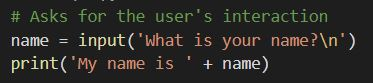Running the example:

``````python .\examples\input.py
``````

The result: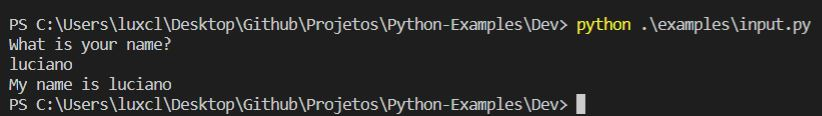### error.py

How a runtime error is shown.
Ex: Multiple math operations are done sequentially until an error occurs.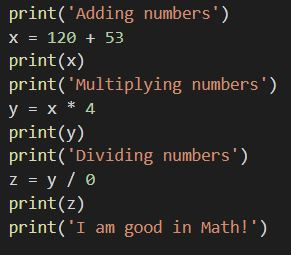Running the example:

``````python .\examples\error.py
``````

The result: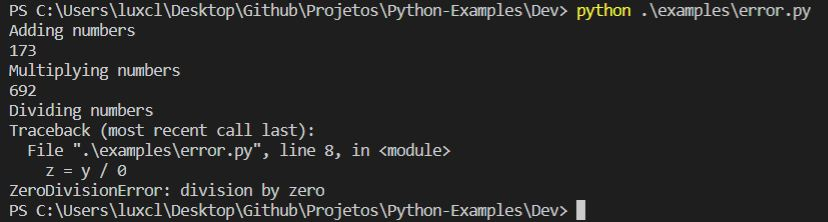An error occured at line 8 because it was not possible to divide by zero.

### strings.py

Strings have many functionalities similar to JavaScript, but with lesser code.
Ex: Using multiple string functions.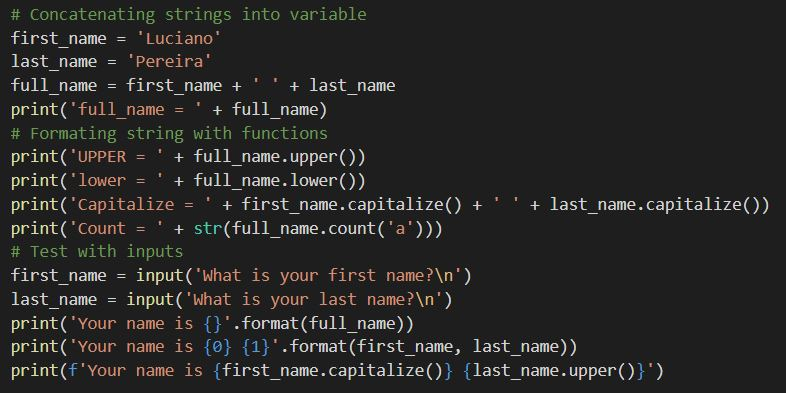Running the example:

``````python .\examples\strings.py
``````

The result: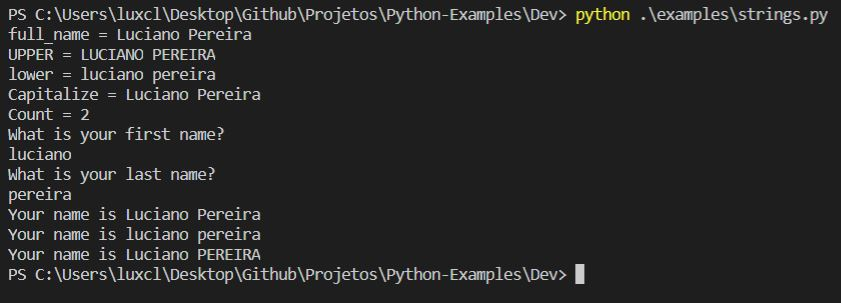## Next

You will learn how to make operations with numbers, dates and how to handle errors.

Categories:
Tags: